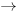# What is Chemical equation? Notes - Class 11

## Class 11: What is Chemical equation? Notes - Class 11

The document What is Chemical equation? Notes - Class 11 is a part of Class 11 category.
All you need of Class 11 at this link: Class 11

A chemical equation is the symbolic representation of a chemical reaction in the form of symbols and formulae, wherein the reactant entities are given on the left-hand side and the product entities on the right-hand side. The coefficients next to the symbols and formulae of entities are the absolute values of the stoichiometric numbers. The first chemical equation was diagrammed by Jean Beguin in 1615.

## Formation of chemical reaction :

A chemical equation consists of the chemical formulas of the reactants (the starting substances) and the chemical formula of the products (substances formed in the chemical reaction). The two are separated by an arrow symbol (                    →              {\displaystyle \rightarrow }, usually read as "yields") and each individual substance's chemical formula is separated from others by a plus sign.

As an example, the equation for the reaction of hydrochloric acid with sodium can be denoted:

2 HCl + 2 Na → 2 NaCl + H
2

This equation would be read as "two HCl plus two Na yields two NaCl and H two." But, for equations involving complex chemicals, rather than reading the letter and its subscript, the chemical formulas are read using IUPAC nomenclature. Using IUPAC nomenclature, this equation would be read as "hydrochloric acid plus sodium yields sodium chloride and hydrogen gas."

This equation indicates that sodium and HCl react to form NaCl and H2. It also indicates that two sodium molecules are required for every two hydrochloric acid molecules and the reaction will form two sodium chloride molecules and one diatomic molecule of hydrogen gas molecule for every two hydrochloric acid and two sodium molecules that react. The stoichiometric coefficients (the numbers in front of the chemical formulas) result from the law of conservation of mass and the law of conservation of charge (see "Balancing Chemical Equation" section below for more information).

The document What is Chemical equation? Notes - Class 11 is a part of Class 11 category.
All you need of Class 11 at this link: Class 11Use Code STAYHOME200 and get INR 200 additional OFF

Track your progress, build streaks, highlight & save important lessons and more!

,

,

,

,

,

,

,

,

,

,

,

,

,

,

,

,

,

,

,

,

,

;